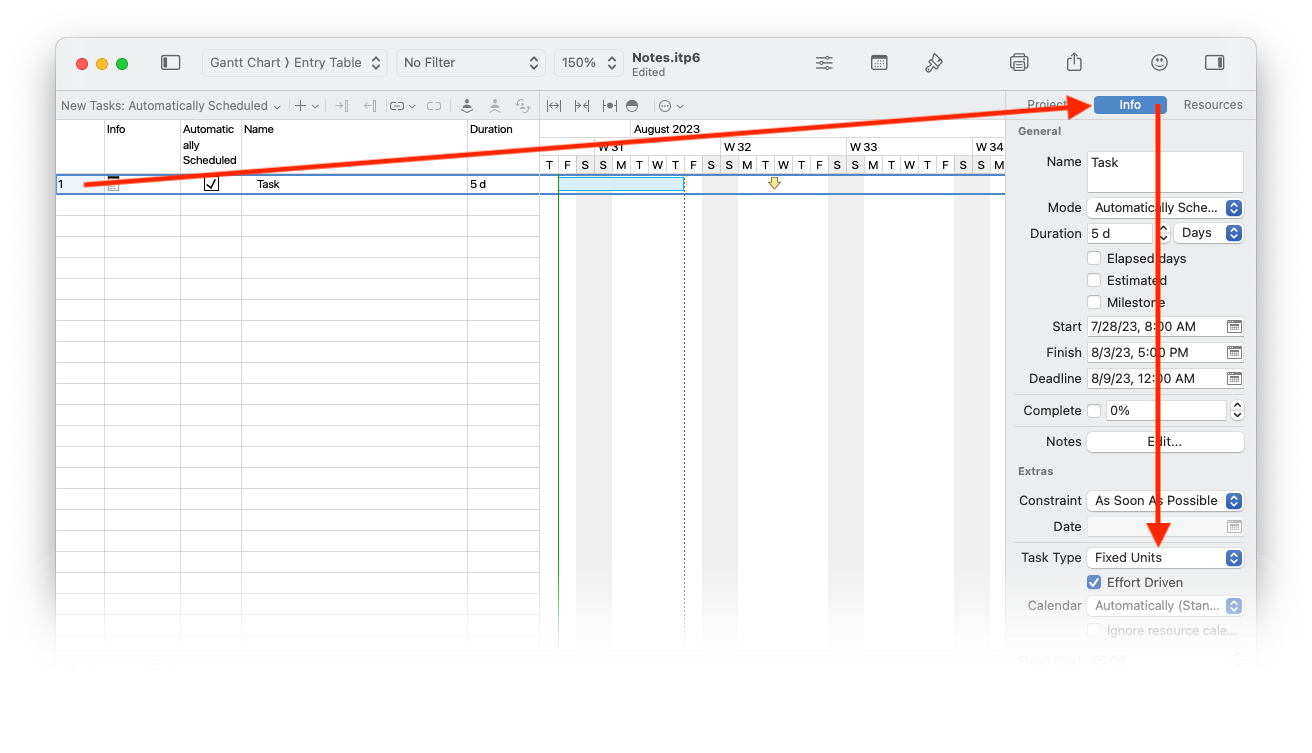If you use this site, you agree to our use of cookies.

#### Help Topics

iTaskX uses one of three task types to calculate the duration of tasks and subsequently their finish dates or their start dates. By default, after you assign a resource, the task is scheduled according to the formula Duration = Work / Units. For any task, you can choose which piece of the equation iTaskX calculates by setting the task type.

Notes

A characterization of a task based on which aspect of the task is fixed and which aspects are variable.
• Finish Date
The date that a task is scheduled to be completed. This date is based on the tasks start date, duration, calendars, predecessor dates, task dependencies and constraints.
• Start Date
The date when a task is scheduled to begin. This date is based on the duration, calendars and constraints of predecessor and successor tasks.

• Fixed Unit
A task in which the assigned resources is a fixed value and any changes to the amount of work, or the tasks duration do not affect the tasks units.
• Fixed Work
A task in which the amount of work is a fixed value and any changes to the task's duration or the number of assigned resources do not affect the tasks work.
• Fixed Duration
A task in which the duration is a fixed value and any changes to the work or the assigned resources, don't affect the tasks duration.

Note
iTaskX uses fixed units by default.

iTaskX uses a scheduling formula that relates the three values of work, duration, and assignment units: Work = Duration x Units

Here’s a handy table to help keep all the moving parts of the scheduling formula in mind:

 Task Type You revise units... You revise duration... You revise work... Fixed Unit Duration is recalculated Work is recalculated Duration is recalculated Fixed Work Duration is recalculated Units are recalculated Duration is recalculated Fixed Duration Work is recalculated Work is recalculated Units are recalculated

Set the task type to calculate duration: